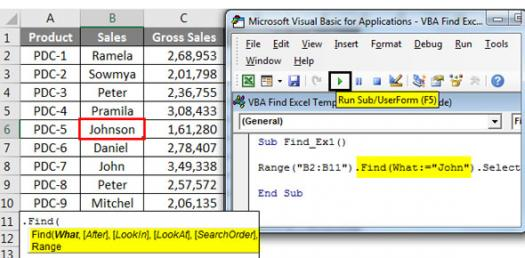# Excel Vba For Actuaries Quiz

Approved & Edited by ProProfs Editorial Team
The editorial team at ProProfs Quizzes consists of a select group of subject experts, trivia writers, and quiz masters who have authored over 10,000 quizzes taken by more than 100 million users. This team includes our in-house seasoned quiz moderators and subject matter experts. Our editorial experts, spread across the world, are rigorously trained using our comprehensive guidelines to ensure that you receive the highest quality quizzes.
| By Actuwaiter
A
Actuwaiter
Community Contributor
Quizzes Created: 2 | Total Attempts: 1,570
Questions: 5 | Attempts: 1,216Settings.

• 1.

### What is a way to code a random number between 0 and 1 in VBA?

• A.

WorksheetFunction.Rand()

• B.

WorksheetFunction.Randbetween(0,100)/10

• C.

WorksheetFunction.Randbetween(0,100)/

• D.

WorksheetFunction.Randbetween(0,100)/100

D. WorksheetFunction.Randbetween(0,100)/100
Explanation
The correct answer is WorksheetFunction.Randbetween(0,100)/100. This code generates a random number between 0 and 100 using the Randbetween function, and then divides it by 100 to scale it down to a number between 0 and 1.

Rate this question:

• 2.

### You want to write "Month" to cell A1.  What code will do this?

• A.

Range("A2").Value = "Month"

• B.

Range("A1") = Month

• C.

Cells(1,1) = "Month"

• D.

Cell(1,1) = "Month"

C. Cells(1,1) = "Month"
Explanation
The correct answer is Cells(1,1) = "Month". This code will write the value "Month" to cell A1.

Rate this question:

• 3.

### Task 2, what is the outer loop? What is the inner loop?

• A.

Outer is simcounter, inner is itemcounter

• B.

Outer is itemcounter, inner is simcounter

• C.

Only itemcounter loop

• D.

Only simcounter loop

A. Outer is simcounter, inner is itemcounter
Explanation
The correct answer is "Outer is simcounter, inner is itemcounter". This means that there are two loops in the code, with the outer loop being controlled by the variable "simcounter" and the inner loop being controlled by the variable "itemcounter". The outer loop will execute its code block first, and then the inner loop will execute its code block for each iteration of the outer loop.

Rate this question:

• 4.

• A.

Functions WarrantyPrice(item,brand) as string

• B.

Functions WarrantyPrice(item,brand) as integer

• C.

Function WarrantyPrice(item) as integer

• D.

Function WarrantyPrice(item,brand) as Double

D. Function WarrantyPrice(item,brand) as Double
Explanation
The correct answer is "Function WarrantyPrice(item,brand) as Double". This is because the function definition includes two parameters, "item" and "brand", and it specifies that the function will return a value of type "Double". This means that the function is expected to take in an item and brand as input and calculate and return a warranty price as a decimal number.

Rate this question:

• 5.

### Task 4, how do you correct this declaration? Dim items As String

• A.

Dim items As Integer

• B.

Dim item As String

• C.

Dim item As Integer

• D.

Delete entire line

B. Dim item As String
Explanation
The correct answer is "Dim item As String" because it replaces the incorrect declaration "Dim items As String" with the correct variable name "item" and the correct data type "String".

Rate this question:

Related TopicsBack to top